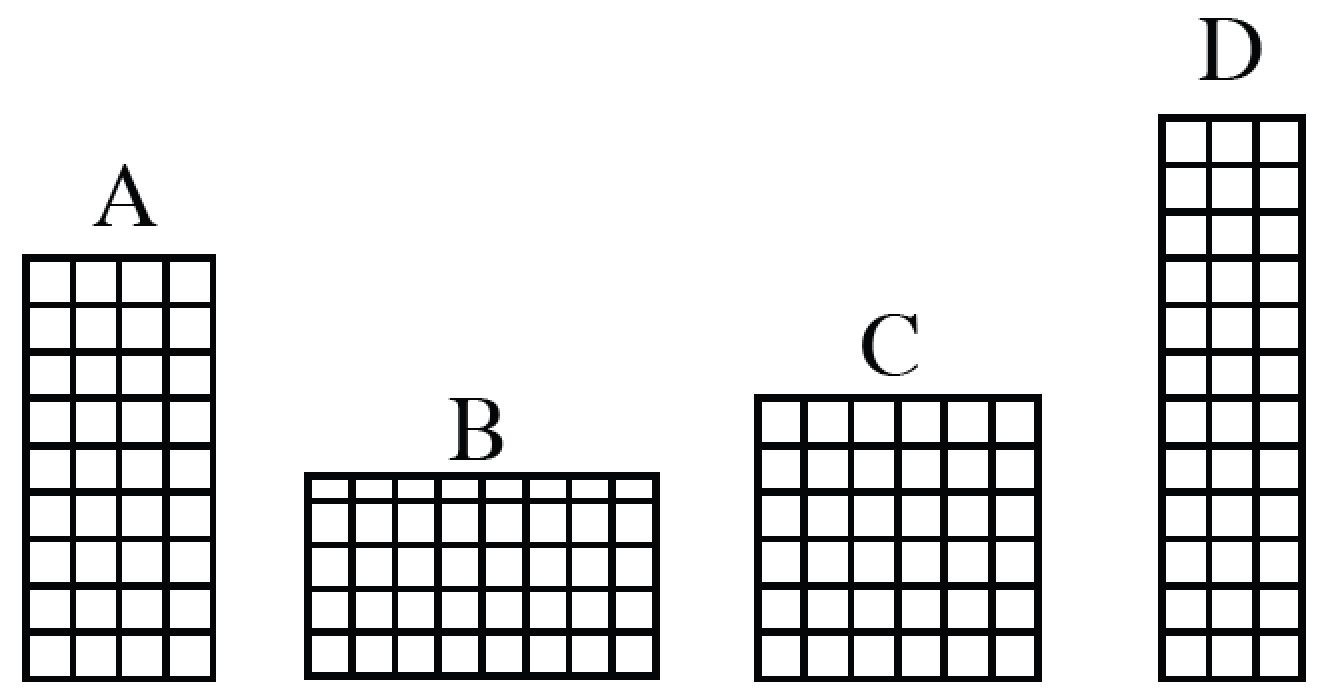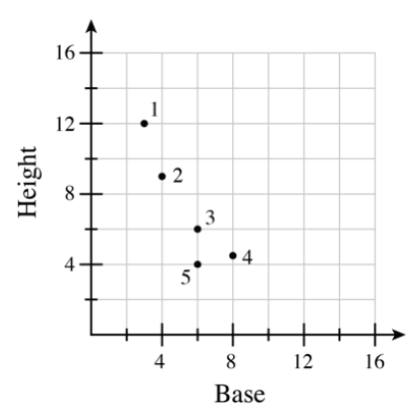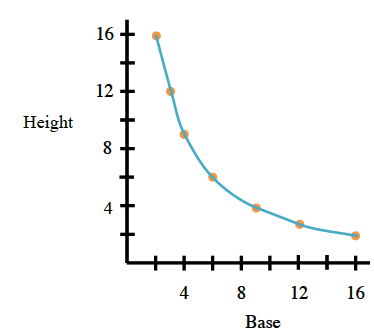### Home > CC3 > Chapter 2 > Lesson 2.1.6 > Problem2-59

2-59.

Cairo wants to create a graph that represents the heights and bases of all rectangles that have an area of $36$ square units. He started by drawing the rectangles A, B, C, and D at right. Examine the dimensions (length and width) of each rectangle.1.Copy the graph at right onto graph paper. Then match the letter of each rectangle above with a point on the graph. Which point is not matched?

Rectangle A has a height of $9$ units and a base of $4$ units. Its corresponding point should be $(4,9)$.

It corresponds to number $2$.

Repeat for each rectangle.

You should find that $5$ is not matched.

1. What are the base, height, and area for the unmatched point?

Point $5$ is $6$ over and $4$ up on the graph. Knowing this information, what are the rectangle's base, height, and area?
Remember that the area is the base multiplied by the height.

2. Why should the unmatched point not be on Cairo's graph?

Check the area of the unmatched rectangle that is represented by point $5$. Does that area meet the qualifications of Cairo's graph that only contains rectangles with areas that are equal to $36$?

3. Find the dimensions of three more rectangles that have areas of $36$ square units. At least one of your examples should have dimensions that are not integers. Place a new point on the graph for each new rectangle you find.

4. Connect all of the points representing an area of $36$ square units. Describe the resulting graph.